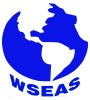### Other Articles by Authors

Talaat Abdelhamid
Meknani Bassem
Asmaa A. Amer
Ahmed Nagah

### Authors and WSEAS

Talaat Abdelhamid
Meknani Bassem
Asmaa A. Amer
Ahmed Nagah# WSEAS Transactions on Mathematics

Print ISSN: 1109-2769
E-ISSN: 2224-2880

Volume 17, 2018

Notice: As of 2014 and for the forthcoming years, the publication frequency/periodicity of WSEAS Journals is adapted to the 'continuously updated' model. What this means is that instead of being separated into issues, new papers will be added on a continuous basis, allowing a more regular flow and shorter publication times. The papers will appear in reverse order, therefore the most recent one will be on top.

Volume 17, 2018

Optimal Control for Systems Described by Semi-linear Parabolic Equations

AUTHORS: Talaat Abdelhamid, Meknani Bassem, Asmaa A. Amer, Ahmed Nagah

ABSTRACT: We study the well-posedness of an optimal control problem described by semi-linear parabolic equation. The control functions are represented by the coefficients λ(u, v) and β(u, v) which appear in the nonlinear part of the state problem and inside the source strength, respectively. These coefficients depend on the control function v. Then, we obtain some necessary optimality conditions for this problem.

KEYWORDS: Optimal control, Quasi-linear parabolic equation, Existence, Uniqueness theorems and necessary optimality conditions

REFERENCES:

 J. L. Lions, Optimal Control of Systems Governed by Partial Differential Equations, Berlin: Springer- Verlag, 1971.

 D. A. Lashin, on the existence of optimal control of temperature regimes, J. of Math. Sciences, 158(2), 2009.

 M. H. Farag, T. A. Talaat and E. M. Kamal, WellPosedness of A Quasilinear Parabolic Optimal Control Problem, IJPAM, (2012).

 M. H. Farag and T. A. Talaat, Combined Exterior Function - Conjugate Gradient Algorithm for A class of Constrained Optimal Control Quasilinear parabolic Systems, ITHEA, 2013.

 N. M. Makhmudov, An Optimal Control Problem for The Schrodinger Equation With A RealValued Factor, Russian Mathematics, Vol.54, No.11, pp.27-35, (2010).

 A. D. Iskenderov and R. K. Tagiev, Optimization problems with controls in coefficients of parabolic equations, Differentsialnye Uravneniya, 19(8), 1983, 1324-1334.

 A. H. Khater, A.B. Shamardan, M.H. Farag and A.H. Abdel-Hamid, Analytical and numerical solutions os a quasilinear parabolic optimal control problem , Journal of Computational and Applied Mathematics, Vol. 95, 1998.

 M. H. Farag, On an Optimal Control problem For a quasilinear parabolic equation, Applicationes Mathematicae, pp.239- 250, 2000. Journal of Computational Mathematics, V. 22(5), 2006, 635–640.

 A. N. Tikhonov and N. Ya. Arsenin, Methods for the solution of incorrectly posed problems, Nauka, Moscow, Russian, 1974.

 R. K. Tagiev, Optimal Control for the coefficient of a Quasilinear parabolic equation, automation and Remote Control, Vol. 70(11), pp.1814- 11826, (2009).

 O. A. Ladyzhenskaya, V. A. Solonnikov and N. N. Ural’tseva, Linear and quasilinear parabolic equations, Nauka, Moscow, Russian,1976.

 O. A. Ladyzhenskaya, Boundary value problems of mathematical physics , Nauka, Moscow, Russian, 1973.

 R. K. Tagiev, On Optimal Control by Coeffi- cients In An Elliptic Equation, Differential Equations, Vol.47( 6), pp. 877-886, (2011).

 M. Goebel, On existence of optimal control, Math. Nuchr., 93, 1979, 67–73.

 K. Glashoff and S. A. Gustafson, Linear Optimization and Approximation, Applied Mathematical Sciences, New York, 1983.

 D. Jiang and T. Abdelhamid, Simultaneous identification of Robin coefficient and heat flux in an elliptic system, International Journal of Computer Mathematics, 94 (1), 1-12, 2017.

 T. Abdelhamid, X. Deng, and R. Chen, A new method for simultaneously reconstructing the space-time dependent Robin coefficient and heat flux in a parabolic system, International Journal of Numerical Analysis and Modeling, 14 (6), pp 893-915, 2017.

 T. Abdelhamid, Simultaneous identification of the spatio-temporal dependent heat transfer coefficient and spatially dependent heat flux using a MCGM in a parabolic system, Journal of Computational and Applied Mathematics, 32, 164- 176, 2017.

 T. Abdelhamid and O. Mumini Omisore, An efficient method for simultaneously reconstructing Robin coefficient and heat flux in an elliptic equation using a MCGM, WSEAS Transactions on Heat And Mass Transfer, 2017.

 T. Abdelhamid, A.H. Elsheikh, and D. Jiang, On the simultaneous reconstruction of heat transfer coefficient and heat flux in the heat conduction problem, under review in Journal of Computational and Applied Mathematics, 2017.

 T. Abdelhamid, A.H. Elsheikh, A. Elazab, S.W. Sharshir, and Ehab S. Selima, D. Jiang, Simultaneous reconstruction of the time-dependent Robin coefficient and heat flux in heat conduction problems, Inverse Problems in Science and Engineering, pp. 1-18, 2017.

WSEAS Transactions on Mathematics, ISSN / E-ISSN: 1109-2769 / 2224-2880, Volume 17, 2018, Art. #9, pp. 58-64